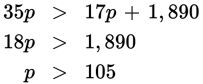# SAT Math Multiple Choice Question 521: Answer and Explanation

### Test Information

Question: 521

11. The chief financial officer of a shoe company calculates that the cost C of producing p pairs of a certain shoe is C = 17p + 1,890. The marketing department wants to sell the shoe for \$35 per pair. The shoe company will make a profit only if the total revenue from selling p pairs is greater than the total cost of producing p pairs. Which of the following inequalities gives the number of pairs of shoes p that the company needs to sell in order to make a profit?

• A. p < 54
• B. p > 54
• C. p < 105
• D. p > 105

Explanation:

D

Difficulty: Medium

Category: Heart of Algebra / Inequalities

Strategic Advice: You could graph the cost function (y = 17p + 1,890) and the revenue function (y = 35x) and try to determine where the revenue function is greater (higher on the graph). However, the numbers are quite large and this may prove to be very time-consuming. Instead, create and solve an inequality comparing revenue and cost.

Getting to the Answer: If the revenue from a single pair of shoes is \$35, then the total revenue from p pairs is 35p. If revenue must be greater than cost, then the inequality must be 35p > 17p + 1,890. Now, solve for p using inverse operations: